# 3. Evaluation

## 3. Evaluation

First, we quantify the performance tradeoffs associated with alternative techniques that support a heterogeneous collection of multi-zone disks. While OLT1, OLT2, HTP, and HetFIXB were quantified using analytic models, HDD was quantified using a simulation study. We conducted numerous experiments analyzing different configurations with different disk models from Quantum and Seagate. Here, we report on a subset of our results in order to highlight the tradeoffs associated with different techniques. In all results presented here, we used the three disk models shown in Table 35.1. Both Barracuda 4LP and 18 provide a 7200 rpm while the Cheetah provides a 10,000 rpm. Moreover, we assumed that all objects in the database require a 4 Mbps bandwidth for their continuous display.

Figures 35.7, 35.8, and 35.9 show the cost per stream as a function of the number of simultaneous displays supported by the system (throughput) for three different configurations. Figure 35.7 shows a system that is installed in 1994 and consists of 10 Barracuda 4LP disks. Figure 35.8 shows the same system two years later when it is extended with 10 Cheetah disks. Finally, Figure 35.9 shows this system in 1998 when it is extended with 10 Barracuda 18 disks. To estimate system cost, we assumed: a) the cost of each disk at the time when they were purchased with no depreciation cost, and, b) the system is configured with sufficient memory to support the number of simultaneous displays shown on the x-axis. We assumed that the cost of memory is \$7/MB, \$5/MB, and \$3/MB in 1994, 1996, and 1998, respectively. Additional memory is purchased at the time of disk purchases in order to support additional users. (Once again, we assume no depreciation of memory.) While one might disagree with our assumptions for computing the cost of the system, note that the focus of this study is to compare the different techniques. As long as the assumptions are kept constant, we can make observations about the proposed techniques and their performance tradeoffs.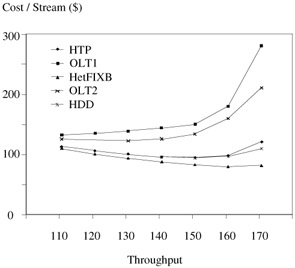Figure 35.7: One Seagate disk model (homogeneous).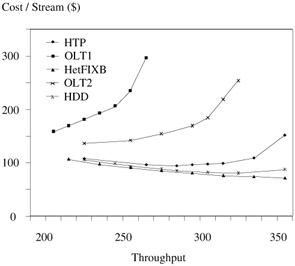Figure 35.8: Two Seagate disk models (heterogeneous).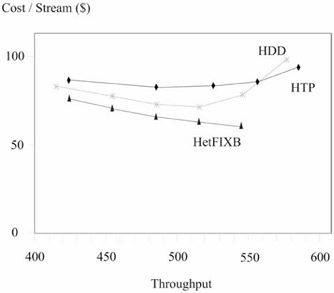Figure 35.9: Three Seagate disk models (heterogeneous).

In these experiments, OLT2 constructed logical zones in order to force all disk models to consist of the same number of zones. This meant that OLT2 eliminated the innermost zone (zone 10) of Barracuda 4LP, splitting the fastest three zones of Cheetah into six zones, and splitting the outermost zone of Barracuda 18 into two. Figure 35.9 does not show OLT1 and OLT2 because: a) their cost per stream is almost identical to that shown in Figure 35.8, and b) we wanted to show the difference between HetFIXB, HDD, and HTP.

Figures 35.7, 35.8, and 35.9 show that HetFIXB is the most cost effective technique; however, it supports fewer simultaneous displays as a function of heterogeneity. For example, with one disk model, it provides a throughput similar to the other techniques. However, with 3 disk models, its maximum throughput is lower than those provided by HDD and HTP. This is dependent on the physical characteristics of the zones because HetFIXB requires the duration of Tscan to be identical for all disk models. This requirement results in fragmentation of the disk bandwidth which in turn limits the maximum throughput of the system. Generally speaking, the greater the heterogeneity, the greater the degree of fragmentation. However, the zone characteristics ultimately decide the degree of fragmentation. One may construct logical zones in order to minimize this fragmentation; see . This optimization is not reported because of strict space limitations imposed by the call for paper. It raises many interesting issues that are not presented here. Regardless, the comparison shown here is fair because our optimizations are applicable to all techniques.

OLT1 provides inferior performance as compared to the other techniques because it wastes a significant percentage of the available disk bandwidth. To illustrate, Figure 35.10 shows the percentage of wasted disk bandwidth for each disk model with each technique when the system is fully utilized (the trend holds true for less than 100% utilization). OLT1 wastes 60% of the bandwidth provided by Cheetah and approximately 30% of Barracuda 18. Most of the wasted bandwidth is attributed to these disks sitting idle. Cheetahs sit idle because they provide a 10,000 rpm as compared to 7200 rpm provided by the Barracudas. Barracuda 4LP and 18 disks sit idle because of their zone characteristics. In passing, while different techniques provide approximately similar cost per performance ratios, each wastes bandwidth in a different manner. For example, both HTP and HetFIXB provide approximately similar cost per performance ratios, HTP wastes 40% of Cheetah's bandwidth while HetFIXB wastes only 20% of the bandwidth provided by this disk model. HTP makes up for this limitation by harnessing a greater percentage of the bandwidth provided by Barracuda 4LP and 18.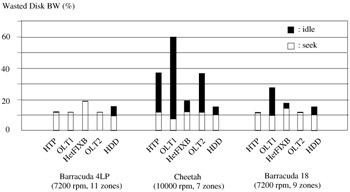Figure 35.10: Wasted disk bandwidth.

Figure 35.11 shows the maximum latency incurred by each technique as a function of the load imposed on the system. In this figure, we have eliminated OLT1 because of its prohibitively high latency. (One conclusion of this study is that OLT1 is not a competitive strategy.) The results show that HetFIXB provides the worst latency while HDD's maximum latency is below 1 second. This is because HetFIXB forces a rigid schedule with a disk zone being activated in an orderly manner (across all disk drives). If a request arrives and the zone containing its referenced block is not active then it must wait until the disk head visits that zone (even if idle bandwidth is available). With HDD, there is no such rigid schedule in place. A request is serviced as soon as there is available bandwidth. Of course, this is at the risk of some requests missing their deadlines. This happens when many requests collide on a single disk drive due to random nature of requests to the disks. In these experiments, we ensured that such occurrences impacted one in a million requests, i.e., a hiccup probability is less than one in a million block requests.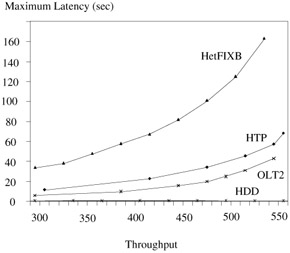Figure 35.11: Maximum startup latency.

OLT2 and HTP provide a better latency as compared to HetFIXB because they construct fewer logical disks [2, 14]. While OLT2 constructs a single logical disk, HTP constructs 10 logical disks, and HetFIXB constructs 30 logical disks. In the worst case scenario (assumed by Figure 35.11), with both HTP and HetFIXB, all active requests collide on a single logical disk (say dbottleneck). A small fraction of them are activated while the rest wait for this group of requests to shift to the next logical disk (in the case of HetFIXB, they wait for one Tscan). Subsequently, another small fraction is activated on dbottleneck. This process is repeated until all requests are activated. Figure 35.11 shows the incurred latency by the last activated request.

With three disk models (Figure 35.9), OLT1 and OLT2 waste more than 80% of disk space, HTP and HDD waste approximately 70% of disk space, while HetFIXB wastes 44% of the available disk space. However, this does NOT mean that HetFIXB is more space efficient than other techniques. This is because the percentage of wasted disk space is dependent on the physical characteristics of the participating disk drives: number of disk models, number of zones per disk, track size of each zone, storage capacity of individual zones and disk drives. For example, with two disk models (Figure 35.8), HetFIXB wastes more disk space when compared with the other techniques.

So far, we have reported experimental results using three real disk models. In the next experiments, we further evaluate the techniques using three imaginary multi-zone disk models that could represent disks in the near future (see Table 35.3). First, we performed similar experiments to quantify the cost per stream. Figure 35.12 shows a system that was installed in 2000 using ten type 0 disks in Table 35.3. Figure 35.13 shows the same system two years later when it is extended with 10 type 1 disks. Finally, Figure 35.14 shows this system in 2004 when it is extended with 10 type 2 disks. We assumed that the cost of memory is \$1/MB, \$0.6/MB, and \$0.35/MB in 2000, 2002, and 2004, respectively.

Table 35.3: Three imaginary disk models and their zone characteristics.

Type 0 30 GB, 2000, \$300

Type 1 80 GB, 2002, \$300

Type 2 150 GB, 2004, \$300

Zone Id

Size (GB)

Rate (Mb/s)

Size (GB)

Rate (Mb/s)

Size (GB)

Rate (Mb/s)

0

10

100

30

150

60

200

1

8

90

12

120

50

150

2

6

80

9

110

40

100

3

4

70

9

100

-

-

4

2

60

8

90

-

-

5

-

-

6

80

-

-

6

-

-

6

70

-

-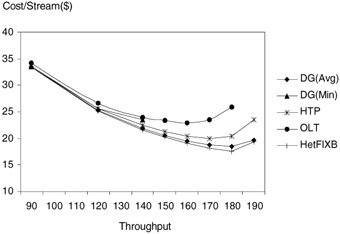Figure 35.12: One disk model, type 0 (homogeneous).Figure 35.13: Two disk models, type 0 and 1 (heterogeneous).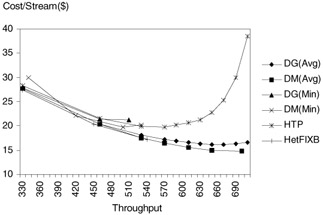Figure 35.14: Three disk models, type 0, 1, 2 (heterogeneous).

Figures 35.12, 35.13, and 35.14 compare all deterministic approaches in non-partitioning techniques. For Disk Grouping (DG) and Merging (DM) techniques, we assumed two different fixed data transfer rates: one with a pessimistic view, the other with an optimistic view. For example, DG(Avg) means disk grouping technique using the average data transfer rate of a multi-zone disk while DG(Min) uses the transfer rate of the slowest zone. Note that there would be no hiccups when the minimum rate is assumed in the above techniques because the technique decides system parameters in a manner that ensures continuous display. However, if the average rate is assumed, hiccups might occur because the active requests might reference the data from the slowest zone, observing a bandwidth lower than the assumed average transfer rate.

Figure 35.12 shows a similar result as in Figure 35.7. HetFIXB and HTP are superior to OLT2. DG(Avg)  also provides a very high throughput with the second lowest cost/stream. However, DG(Min) supports only 140 at maximum because of the lowered disk bandwidth to guarantee a continuous display. Figure 35.13 and 14 also show a similar trend. DG(Avg) and DM(Avg) provide the best cost/stream being followed by HetFIXB and HTP.

Figure 35.15 shows the percentage of wasted disk space with each technique. It is important to note that the obtained results are dependent on the characteristics of the assumed disk drives, namely the discrepancy between the bandwidth and storage ratios of different disk models. For example, the bandwidth of type 2 disk is twice that of type 0 disk. However, the storage capacity of type 2 disk is five times that of type 0 disk. Because all techniques construct logical blocks based on the disk bandwidth ratio, they waste a considerable amount of storage provided by type 1 and 2 disks.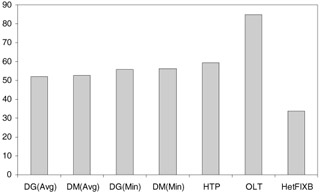Figure 35.15: Wasted disk space with three disk models.

For single model, DG(Avg) is equal to DM(Avg) and DG(Min) is equal to DM(Min).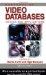Handbook of Video Databases: Design and Applications (Internet and Communications)
ISBN: 084937006X
EAN: 2147483647
Year: 2003
Pages: 393

flylib.com © 2008-2017.
If you may any questions please contact us: flylib@qtcs.net